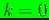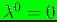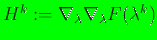# Numerical Implementation

On considering the dual formulation, the solution for the Lagrange multipliers can be written as [16,17](5)

whereis the optimal solution that is desired. Sinceis strictly convex in the interior of, convex optimization algorithms (for example, Newton's method and families of gradient descent) are a natural choice. The steps in these algorithm are:

1. Start with iteration counter. The initial guessand letbe the desired convergence tolerance. For the convergence tolerance,-is suitable (see sample input data files in the tests sub-directory);
2. Compute(gradient of) and(Hessian of);
3. Determine a suitable search direction,. For steepest descent,and for Newton's method,(matrix-vector notation) are used;
4. Update:, whereis the step size. For steepest descent, a variable step size (line search) algorithm, which is presented in Reference , is used to determine, and for Newton's method (damped or guarded), the line search is used to set the step size if the error is greater thanand otherwise,is used;
5. Check convergence: if, increment the iteration counter,, and goto 2, else continue;
6. Setand compute the max-ent basis functions using Eq. (4).

N. Sukumar